I. 神经网络历史

1943年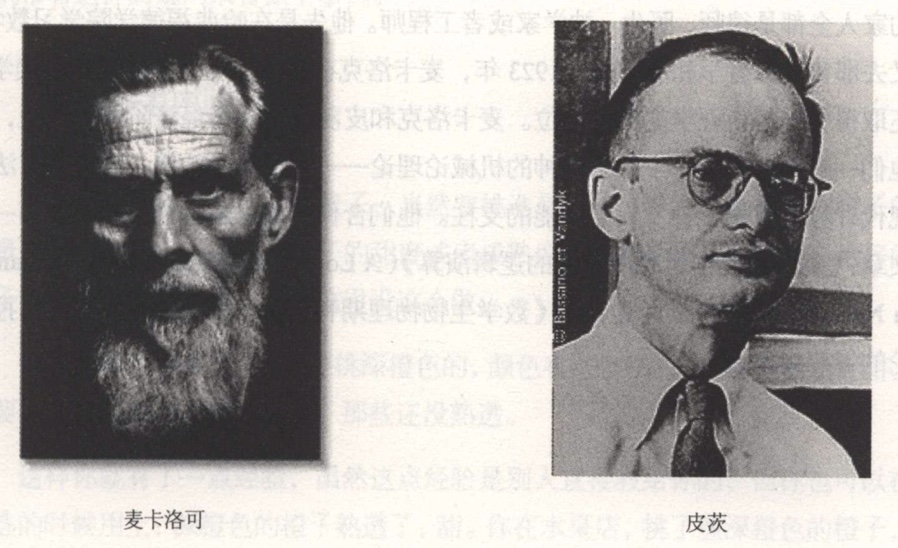1947年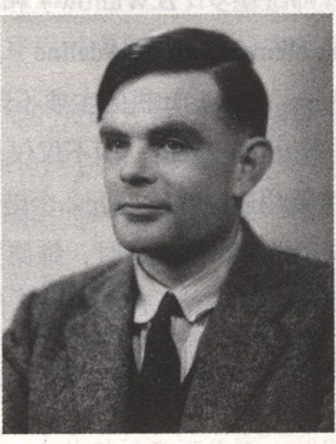人工智能流派

1. 自顶向下的逻辑和符号系统(通过狭义统计学预设和反馈来达成)
2. 自底向上的仿造大脑来达成(模拟大脑中的神经网络)

1952年1982年2015年5月28日LeCun、Bengio、Hinton在Nature上发布一篇名为Deep Learning的综述并开设Deep Learning专栏，涉及概率机器学习、强化机器学习、机器人等多方面，卷积神经网络(CNN)改进再应用: 勒丘恩(Lecun): 第一个把BP算法用在CNN上，完善CNN使其可以真正应用上工作，1998年后近20年CNN第一推动者

2016年3月10日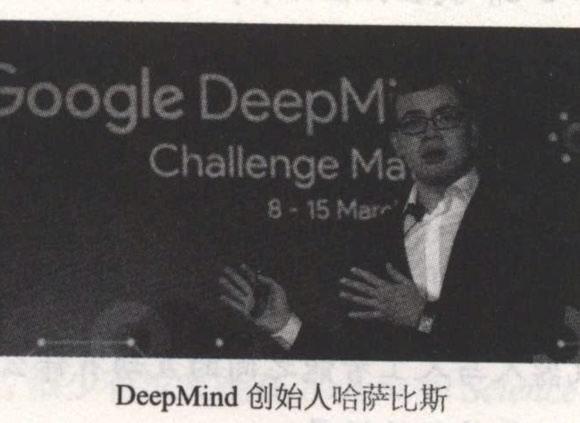II. 从买橙子说起

• 什么橙子最甜? 个头大的比较甜
• 另一种品种，中小的、浅色的最甜: 迁移学习
• 表妹不在乎甜不甜，多汁就好，捏起来越有弹性汁越多: 优化目标变化

编程思路

• 下发配置
• if...then

机器学习思路

• Traning Data: 记录橙子属性和类型的关系
• Feature: 颜色、大小、形状、场地
• Output Variables: 甜度、成熟度、多汁度

III. 大脑学习过程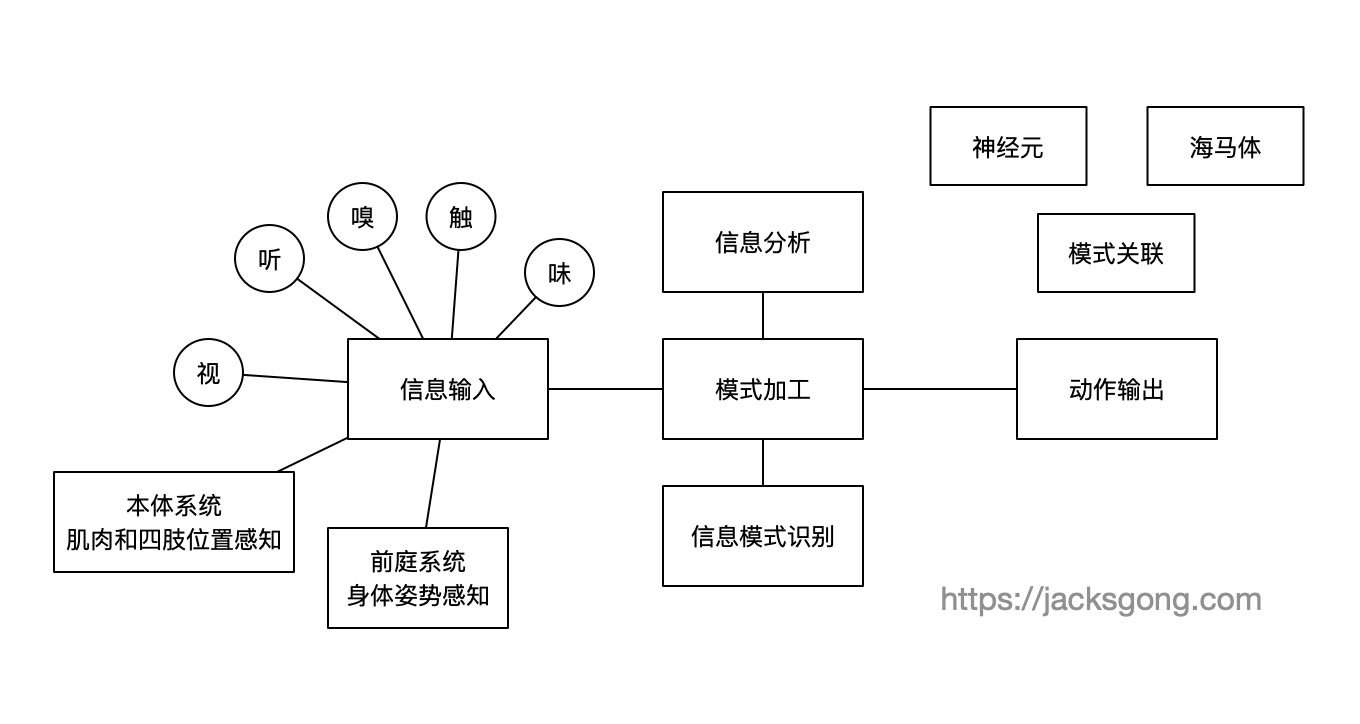生物意义上的神经元

神经元是如何工作的?• 树突: 接收其他神经元的信息并传至胞体
• 轴突: 把冲动传至远处，传给另一个神经元的树突或肌肉与腺体
• 细胞体: 进行复杂的化学变化，确定是否需要对轴突产生输出

• 当刺激强度未达到某一阀值时，神经冲动不会发生
• 当刺激强度达到该阀值时，神经冲动发生并能瞬时达到最大强度，此后刺激强度即使再继续加强或减弱，已诱发的冲动强度也不再发生变化

• 感觉神经元，作为传入神经元将兴奋传至脊髓和大脑
• 运动神经元，作为传出神经元将通过兴奋引起肌肉与腺体活动
• 联络神经元，将传入神经元与传出神经元联络起来组成复杂的网络，多存在于脑和脊髓里

神经网络的自组织III. 构造神经网络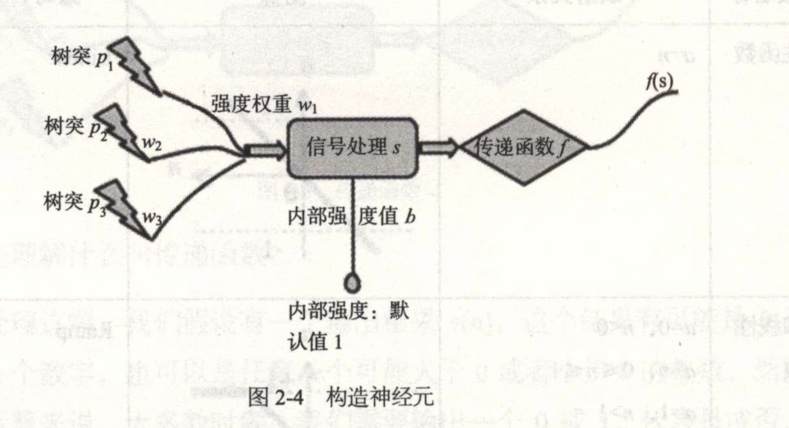• 拥有多个树突p，每个树突传入有不同的权重w
• 信息处理将所有树突的值累加起来在加上内部默认强度b: $s=p1w1+p2w2+…+pnwn +b$
• 传到轴突时，通过传递函数进行”化学反应” $f(s)$，然后输出结果感知机

$$w_1=w_2=1$$
$$b=0$$

$$s=1*1+1*1+0=2$$
$$f(2) = 1$$

$$s = -1*1 + -1*1 + 0 = -2$$
$$f(-2) = 0$$

感知机的学习

$$w_1=1, w_2=-1, b=0$$

$$s = 1*1 + 1*(-1) + 0 = 0$$
$$f(0) = 0$$

$$w(new)=w(old)+e$$
$$b(new)=b(old)+b$$

$$e=1-0=1$$

$$w1=1+1=2$$
$$w2=-1+1=0$$

$$b=0+1=1$$

$$s=1*2+1*0+1=3$$
$$f(3)=1$$

$$s=-1*2+(-1)*0+1=-1$$
$$f(-1)=0$$